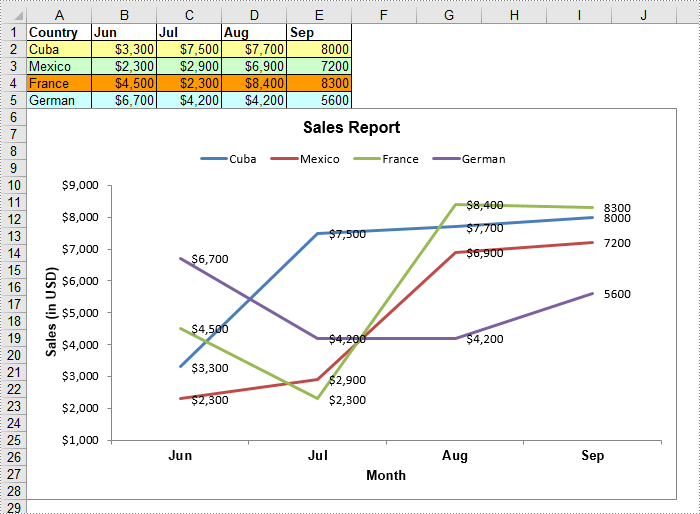# C#/VB.NET: Create a Line Chart in Excel

A line chart, also known as a line graph, is a type of chart that displays information as a series of data points connected by straight line segments. It is generally used to show the changes of information over a period of time, such as years, months or days. In this article, you will learn how to create a line chart in Excel in C# and VB.NET using Spire.XLS for .NET.

### Install Spire.XLS for .NET

To begin with, you need to add the DLL files included in the Spire.XLS for .NET package as references in your .NET project. The DLL files can be either downloaded from this link or installed via NuGet.

`PM> Install-Package Spire.XLS`

### Create a Line Chart in Excel using C# and VB.NET

The following are the main steps to create a line chart:

• Create an instance of Workbook class.
• Get the first worksheet by its index (zero-based) though Workbook.Worksheets[sheetIndex] property.
• Add some data to the worksheet.
• Set data range for the chart through Chart.DataRange property.
• Set position, title, category axis title and value axis title for the chart.
• Loop through the data series of the chart, show data labels for the data points of each data series by setting the ChartSerie.DataPoints.DefaultDataPoint.DataLabels.HasValue property as true.
• Set the position of chart legend through Chart.Legend.Position property.
• Save the result file using Workbook.SaveToFile() method.
• C#
• VB.NET
```using Spire.Xls;
using Spire.Xls.Charts;
using System.Drawing;

namespace CreateLineChart
{
class Program
{
static void Main(string[] args)
{
//Create a Workbook instance
Workbook workbook = new Workbook();

//Get the first worksheet
Worksheet sheet = workbook.Worksheets;
//Set sheet name
sheet.Name = "Line Chart";
//Hide gridlines
sheet.GridLinesVisible = false;

//Add some data to the the worksheet
sheet.Range["A1"].Value = "Country";
sheet.Range["A2"].Value = "Cuba";
sheet.Range["A3"].Value = "Mexico";
sheet.Range["A4"].Value = "France";
sheet.Range["A5"].Value = "German";

sheet.Range["B1"].Value = "Jun";
sheet.Range["B2"].NumberValue = 3300;
sheet.Range["B3"].NumberValue = 2300;
sheet.Range["B4"].NumberValue = 4500;
sheet.Range["B5"].NumberValue = 6700;

sheet.Range["C1"].Value = "Jul";
sheet.Range["C2"].NumberValue = 7500;
sheet.Range["C3"].NumberValue = 2900;
sheet.Range["C4"].NumberValue = 2300;
sheet.Range["C5"].NumberValue = 4200;

sheet.Range["D1"].Value = "Aug";
sheet.Range["D2"].NumberValue = 7700;
sheet.Range["D3"].NumberValue = 6900;
sheet.Range["D4"].NumberValue = 8400;
sheet.Range["D5"].NumberValue = 4200;

sheet.Range["E1"].Value = "Sep";
sheet.Range["E2"].NumberValue = 8000;
sheet.Range["E3"].NumberValue = 7200;
sheet.Range["E4"].NumberValue = 8300;
sheet.Range["E5"].NumberValue = 5600;

//Set font and fill color for specified cells
sheet.Range["A1:E1"].Style.Font.IsBold = true;
sheet.Range["A2:E2"].Style.KnownColor = ExcelColors.LightYellow;
sheet.Range["A3:E3"].Style.KnownColor = ExcelColors.LightGreen1;
sheet.Range["A4:E4"].Style.KnownColor = ExcelColors.LightOrange;
sheet.Range["A5:E5"].Style.KnownColor = ExcelColors.LightTurquoise;

//Set cell borders
sheet.Range["A1:E5"].Style.Borders[BordersLineType.EdgeTop].Color = Color.FromArgb(0, 0, 128);
sheet.Range["A1:E5"].Style.Borders[BordersLineType.EdgeTop].LineStyle = LineStyleType.Thin;
sheet.Range["A1:E5"].Style.Borders[BordersLineType.EdgeBottom].Color = Color.FromArgb(0, 0, 128);
sheet.Range["A1:E5"].Style.Borders[BordersLineType.EdgeBottom].LineStyle = LineStyleType.Thin;
sheet.Range["A1:E5"].Style.Borders[BordersLineType.EdgeLeft].Color = Color.FromArgb(0, 0, 128);
sheet.Range["A1:E5"].Style.Borders[BordersLineType.EdgeLeft].LineStyle = LineStyleType.Thin;
sheet.Range["A1:E5"].Style.Borders[BordersLineType.EdgeRight].Color = Color.FromArgb(0, 0, 128);
sheet.Range["A1:E5"].Style.Borders[BordersLineType.EdgeRight].LineStyle = LineStyleType.Thin;

//Set number format
sheet.Range["B2:D5"].Style.NumberFormat = "\"\$\"#,##0";

//Add a line chart to the worksheet

//Set data range for the chart
chart.DataRange = sheet.Range["A1:E5"];

//Set position of the chart
chart.LeftColumn = 1;
chart.TopRow = 6;
chart.RightColumn = 11;
chart.BottomRow = 29;

//Set and format chart title
chart.ChartTitle = "Sales Report";
chart.ChartTitleArea.IsBold = true;
chart.ChartTitleArea.Size = 12;

//Set and format category axis title
chart.PrimaryCategoryAxis.Title = "Month";
chart.PrimaryCategoryAxis.Font.IsBold = true;
chart.PrimaryCategoryAxis.TitleArea.IsBold = true;

//Set and format value axis title
chart.PrimaryValueAxis.Title = "Sales (in USD)";
chart.PrimaryValueAxis.HasMajorGridLines = false;
chart.PrimaryValueAxis.TitleArea.TextRotationAngle = -90;
chart.PrimaryValueAxis.MinValue = 1000;
chart.PrimaryValueAxis.TitleArea.IsBold = true;

//Loop through the data series of the chart
foreach (ChartSerie cs in chart.Series)
{
cs.Format.Options.IsVaryColor = true;
//Show data labels for data points
cs.DataPoints.DefaultDataPoint.DataLabels.HasValue = true;
}

//Set position of chart legend
chart.Legend.Position = LegendPositionType.Top;

//Save the result file
workbook.SaveToFile("LineChart.xlsx", ExcelVersion.Version2016);
}
}
}```### Apply for a Temporary License

If you'd like to remove the evaluation message from the generated documents, or to get rid of the function limitations, please request a 30-day trial license for yourself.